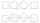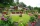A photograph

A photograph will stick to a white square letter with a x cm length. The photo is 3/4 x cm long and 20 cm wide than the width of the paper. The surface of the remaining paper surrounding the photograph is 990 cm2. Find the size of paper and photo.

Result

a =  39.846 cm
b =  29.885 cm
c =  20 cm

Solution:Checkout calculation with our calculator of quadratic equations.Leave us a comment of example and its solution (i.e. if it is still somewhat unclear...):Be the first to comment!To solve this example are needed these knowledge from mathematics:

Looking for help with calculating roots of a quadratic equation? Do you have a linear equation or system of equations and looking for its solution? Or do you have quadratic equation?

Next similar examples:

1. RectangleCalculate area of the rectangle if its length is 12 cm longer than its width and length is equal to the square of its width.
2. Square and rectangleCalculate the side of a square which content area equals area of the rectangle having a length of 3 cm greater and by 2 cm smaller than the side of the square.
3. Rectangle - area, perimeterThe area of a rectangular field is equal to 300 square meters. Its perimeter is equal to 70 meters. Find the length and width of this rectangle.
4. Square to rectangleWhat is the ratio of the area of a square of side x to the area of a rectangle of a rectangle of width 2 x and length 3
5. Square root 2If the square root of 3m2 +22 and -x = 0, and x=7, what is m?
6. Solve equationsolve equation: ?
7. Rectangular garden 2A farmer bought 600 m of wire for the fence. He wants to use it to besiege a rectangular garden with a surface of 16875 m2. Calculate the size of the garden.
8. A rectangular patioA rectangular patio measures 20 ft by 30 ft. By adding x feet to the width and x feet to the length, the area is doubled. Find the new dimensions of the patio.
9. The lengthThe length of a rectangle is 6 meters less than twice the width. If the area of the rectangle is 216 meters, find the dimensions of the rectangle.
10. TrapeziumThe lengths of a parallel sides of a trapezium are (2x+3) and (x+8) and the distance between them is (x+4). if the area of the trapezium is 590 , find the value of x.
11. RootsDetermine the quadratic equation absolute coefficient q, that the equation has a real double root and the root x calculate: ?
12. DiscriminantDetermine the discriminant of the equation: ?
13. Solve 3Solve quadratic equation: (6n+1) (4n-1) = 3n2
14. Evaluation of expressionsIf a2-3a+1=0, find (i)a2+1/a2 (ii) a3+1/a3
15. Theorem proveWe want to prove the sentence: If the natural number n is divisible by six, then n is divisible by three. From what assumption we started?Find the roots of the quadratic equation: 3x2-4x + (-4) = 0.Equation ? has one root x1 = 8. Determine the coefficient b and the second root x2.